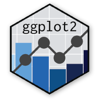Show Sidebar Hide Sidebar# Axis Text in ggplot2

How to modify axis titles in R and ggplot2.

### New to Plotly?

Plotly's R library is free and open source!
You can set up Plotly to work in online or offline mode.
We also have a quick-reference cheatsheet (new!) to help you get started!

### Version Check

Version 4 of Plotly's R package is now available!
Check out this post for more information on breaking changes and new features available in this version.

library(plotly)
packageVersion('plotly')

##  '4.9.1'


### Axis Text Size

library(plotly)
set.seed(123)

df <- diamonds[sample(1:nrow(diamonds), size = 1000),]

p <- ggplot(df, aes(carat, price, color = color, alpha = cut)) +
geom_point() +
theme(axis.text.x = element_text(colour = "#ff6666", size = 20),
axis.text.y = element_text(colour = "#668cff", size = 20))

p <- ggplotly(p)

p


### Axis Text Blank

library(plotly)
set.seed(123)

df <- diamonds[sample(1:nrow(diamonds), size = 1000),]

p <- ggplot(df, aes(carat, price, color = cut)) +
geom_point() +
theme(axis.text = element_blank())

p <- ggplotly(p)

p


### Vertical Text

library(plotly)

lab <- paste("Vertical Label", c(1, 2, 3, 4, 5))

ds <- data.frame(x = sample(lab, size = 1000, replace = T),
y = sample(LETTERS[1:5], size = 1000, replace = T))

p <- ggplot(ds, aes(x = x, fill = y)) +
geom_bar() +
theme(axis.text.x = element_text(angle = 90)) +
ggtitle("Vertical Axis Labels")

p <- ggplotly(p)

p


### Angled Text

library(plotly)

lab <- paste("Angle Label", c(1, 2, 3, 4, 5))

ds <- data.frame(x = sample(lab, size = 1000, replace = T),
y = sample(LETTERS[1:5], size = 1000, replace = T))

p <- ggplot(ds, aes(x = x, fill = y)) +
geom_bar() +
theme(axis.text.x = element_text(angle = 45)) +
ggtitle("Angle Axis Labels")

p <- ggplotly(p)

p


Inspired by ggplot2 documentation